Demo problem: A one-dimensional eigenproblem

In this document, we demonstrate how to solve a 1D eigenproblem (eigenvalues of the 1D Laplace operator in a bounded domain) by creating custom elements. The tutorial is similar in spirit to the quick guide but far less detailed.

 One-dimensional model eigenvalue problem Solve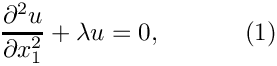in the domain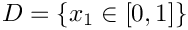, with homogeneous Dirichlet boundary conditions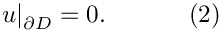An eigenfunction must be non-zero by definition and so the exact solutions are given by the countably infinite set: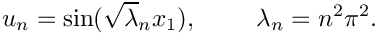We provide a detailed discussion of the driver code harmonic.cc which solves the problem for the first four eigenvalues.

In oomph-lib all eigenproblems are solved by using interfaces to third-party libraries. The default is LAPACK's (direct) QZ solver which is included with the library. An interface is also provided to the serial version of ARPACK, an iterative solver, but we are not allowed to redistribute the source code, so it must be downloaded separately. That said, much of the functionality of ARPACK has been superseded by the ANASAZI solver that is part of The Trilinos Project. Thus, if you want to solve small problems, use the default QZ solver; for larger problems, we recommend using ANASAZI.

# Overview of the theory

A discrete (linear) eigenproblem takes the form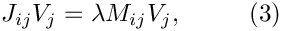where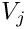is the vector of discrete unknowns,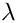is the eigenvalue, and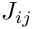and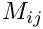are two matrices:(often denotedin the literature) is known as the stiffness matrix andis termed the mass matrix. The third-party libraries mentioned above all solve systems of the form (3), and within oomph-lib we must simply provide the required mass and stiffness matrices.

## Linear Stability Theory

In many cases, eigenproblems arise from linear stability analyses of steady solutions to our set of nonlinear residuals. For example, if we have a time-dependent system of equations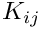then the residual formulation isand steady solutions,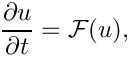are found by setting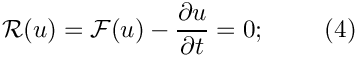. Note that in a nonlinear system there may be many possible steady solutions for the same set of parameter values.

A linear stability analysis proceeds by assuming that the steady solution is perturbed by a small amount, so that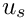, where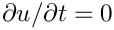. If we substitute this expression into the governing equation (4) and neglect terms that involve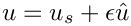(on the assumption that they are really small) then we obtain the equation: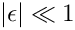Note that we have used a Taylor expansion of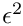, so we are assuming that such an operation "makes sense". The solutionis a steady state, so by definition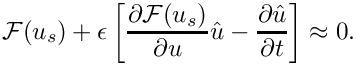and then we are left with the linear equation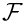The Jacobianis exactly the same as that required by the Newton method when solving the steady set of equations,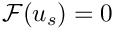The general solution of equation (5) takes the form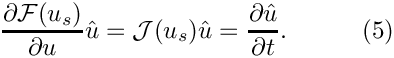because the only function that is proportional to its derivative is the exponential. The necessary functional form of the solution means that equation (5) becomes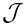which is an eigenproblem in which the mass "matrix" is the identity. Thus, in order to assess the stability of a solution to our nonlinear system we must solve an eigenproblem using the Jacobian matrix, which is why we used the notationrather thanin equation (3). The solution is said to be linearly stable if the real part of all eigenvalues is negative because then the perturbation decays as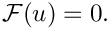, but it is linearly unstable if even a single eigenvalue has positive real part. Note that in even more general equations the mass "matrix" is not necessarily the identity.

## Weak formulation of the problem

If we wish to solve the problem (1) using finite elements, we must first derive the weak form, which follows from the standard procedure of multiplication by a test function and integration by parts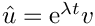Note that the boundary terms are neglected as a consequence of the Dirichlet boundary conditions.

If we expand the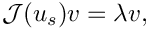in terms of known basis functions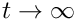and use the same basis functions as our test functions (Galerkin method), then the weak form becomes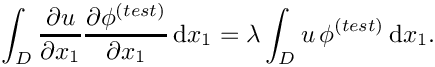On comparison with equation (3), we identify the Jacobian and mass matrices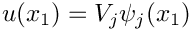# Implementation

In oomph-lib, eigenproblems are formulated on an element-by-element basis in a similar way to standard problems. Eigenproblems make use of the function

GeneralisedElement::get_jacobian_and_mass_matrix(
Vector<double> &residuals,
DenseMatrix<double> &jacobian,
DenseMatrix<double> &mass_matrix);

where the Jacobian is the matrix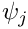and the mass matrix is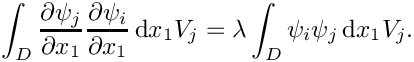in equation (3). The residuals need not be returned when solving an eigenproblem, but the interface is chosen so that linear stability of solutions to the nonlinear system can easily be calculated without reformulating the problem.

Once again, to avoid reinitialisation in multi-physics problems, the helper function

GeneralisedElement::fill_in_contribution_to_jacobian_and_mass_matrix(
Vector<double> &residuals,
DenseMatrix<double> &jacobian,
DenseMatrix<double> &mass_matrix);

is used and this will be overloaded in our custom elements below.

## Creating the elements

For generality, we implement the mathematics to assemble contributions to the Jacobian and mass matrices defined in equations (6) in the class HarmonicEquations that inherits from FiniteElement. This construction mirrors that in our standard equation classes and allows a clean separation between the equations and the choice of basis function.

/// A class for all elements that solve the simple one-dimensional
/// eigenvalue problem
/// \f[
/// \frac{\partial^2 u}{\partial x_i^2} + \lambda u = 0
/// \f]
/// These elements are very closely related to the Poisson
/// elements and could inherit from them. They are here developed
/// from scratch for pedagogical purposes.
/// This class contains the generic maths. Shape functions, geometric
/// mapping etc. must get implemented in derived class.
//================================================================
class HarmonicEquations : public virtual FiniteElement
{
public:
/// Empty Constructor
HarmonicEquations() {}

The unknowns that represent the discretised eigenfunction are assumed to be stored at the nodes.

/// Access function: Eigenfunction value at local node n
/// Note that solving the eigenproblem does not assign values
/// to this storage space. It is used for output purposes only.
virtual inline double u(const unsigned& n) const
{return nodal_value(n,0);}

The class contains functions to output the eigenfunction; interpolate the nodal unknown; and provides interfaces for the shape functions and their derivatives. The key function is fill_in_contribution_to_jacobian_and_mass_matrix which implements the calculation of the equations (6). The residuals vector is not filled in and does not need to be unless we also wish to solve an associated (non-eigen) problem.

void fill_in_contribution_to_jacobian_and_mass_matrix(
Vector<double> &residuals,
DenseMatrix<double> &jacobian, DenseMatrix<double> &mass_matrix)
{
//Find out how many nodes there are
unsigned n_node = nnode();
//Set up memory for the shape functions and their derivatives
Shape psi(n_node);
DShape dpsidx(n_node,1);
//Set the number of integration points
unsigned n_intpt = integral_pt()->nweight();
//Integers to store the local equation and unknown numbers
int local_eqn=0, local_unknown=0;
//Loop over the integration points
for(unsigned ipt=0;ipt<n_intpt;ipt++)
{
//Get the integral weight
double w = integral_pt()->weight(ipt);
//Call the derivatives of the shape and test functions
double J = dshape_eulerian_at_knot(ipt,psi,dpsidx);
//Premultiply the weights and the Jacobian
double W = w*J;
//Assemble the contributions to the mass matrix
//Loop over the test functions
for(unsigned l=0;l<n_node;l++)
{
//Get the local equation number
local_eqn = u_local_eqn(l);
/*IF it's not a boundary condition*/
if(local_eqn >= 0)
{
//Loop over the shape functions
for(unsigned l2=0;l2<n_node;l2++)
{
local_unknown = u_local_eqn(l2);
//If at a non-zero degree of freedom add in the entry
if(local_unknown >= 0)
{
jacobian(local_eqn,local_unknown) += dpsidx(l,0)*dpsidx(l2,0)*W;
mass_matrix(local_eqn, local_unknown) += psi(l)*psi(l2)*W;
}
}
}
}
}
} //end_of_fill_in_contribution_to_jacobian_and_mass_matrix

The shape functions are specified in the QHarmonicElement class that inherits from our standard one-dimensional Lagrange elements QElement<1,NNODE_1D> as well as HarmonicEquations. The number of unknowns (one) is specified and the output functions and shape functions are overloaded as required: the output functions are specified in the HarmonicEquations class, whereas the shape functions are provided by the QElement<1,NNODE_1D> class.

template <unsigned NNODE_1D>
class QHarmonicElement : public virtual QElement<1,NNODE_1D>,
public HarmonicEquations
{
public:
/// Constructor: Call constructors for QElement and
/// Poisson equations
QHarmonicElement() : QElement<1,NNODE_1D>(), HarmonicEquations() {}
/// Required # of values' (pinned or dofs)
/// at node n
inline unsigned required_nvalue(const unsigned &n) const {return 1;}
/// Output function overloaded from HarmonicEquations
void output(ostream &outfile)
{HarmonicEquations::output(outfile);}
/// Output function overloaded from HarmonicEquations
void output(ostream &outfile, const unsigned &Nplot)
{HarmonicEquations::output(outfile,Nplot);}
protected:
/// Shape, test functions & derivs. w.r.t. to global coords. Return Jacobian.
inline double dshape_eulerian(const Vector<double> &s,
Shape &psi,
DShape &dpsidx) const
{return QElement<1,NNODE_1D>::dshape_eulerian(s,psi,dpsidx);}
/// Shape, test functions & derivs. w.r.t. to global coords. at
/// integration point ipt. Return Jacobian.
inline double dshape_eulerian_at_knot(const unsigned& ipt,
Shape &psi,
DShape &dpsidx) const
{return QElement<1,NNODE_1D>::dshape_eulerian_at_knot(ipt,psi,dpsidx);}
}; //end_of_QHarmonic_class_definition
QHarmonicElement<NNODE_1D> elements are 1D Elements with NNODE_1D nodal points that are used to solve...
Definition: harmonic.cc:224
QHarmonicElement()
Constructor: Call constructors for QElement and Poisson equations.
Definition: harmonic.cc:230
double dshape_eulerian(const Vector< double > &s, Shape &psi, DShape &dpsidx) const
Shape, test functions & derivs. w.r.t. to global coords. Return Jacobian.
Definition: harmonic.cc:248
double dshape_eulerian_at_knot(const unsigned &ipt, Shape &psi, DShape &dpsidx) const
Shape, test functions & derivs. w.r.t. to global coords. at integration point ipt....
Definition: harmonic.cc:256
unsigned required_nvalue(const unsigned &n) const
Required # of ‘values’ (pinned or dofs) at node n.
Definition: harmonic.cc:234
void output(ostream &outfile)
Definition: harmonic.cc:237

# The driver code

In order to solve the 1D eigenproblem using oomph-lib, we represent the mathematical problem defined by equations (1) and (2) in a specific Problem object, HarmonicProblem, which is templated by the element type and the eigensolver. We use QHarmonicElement<3>, a quadratic element and our three different eigensolvers, including timing statements to compare the three approaches. Note that if you do not have Trilinos or ARPACK installed then only the LAPACK_QZ solver will do anything.

The problem class takes a single argument corresponding to the number of elements used to discretise the domain and contains a member function solve that takes an integer used for documentation purposes.

unsigned n_element=100; //Number of elements
clock_t t_start1 = clock();
//Solve with ARPACK
{
problem(n_element);
std::cout << "Matrix size " << problem.ndof() << std::endl;
problem.solve(1);
}
clock_t t_end1 = clock();
1D Harmonic problem in unit interval.
Definition: harmonic.cc:269

The same problem is then solved with a different solver

clock_t t_start2 = clock();
//Solve with LAPACK_QZ
{
problem(n_element);
problem.solve(2);
}
clock_t t_end2 = clock();

and again with the Trilinos ANASAZI solver if it is installed.

# The problem class

The HarmonicProblem is derived from oomph-lib's generic Problem class and the specific element type and eigensolver are specified as template parameters to make it easy for the "user" to change either of these from the driver code.

//==start_of_problem_class============================================
/// 1D Harmonic problem in unit interval.
//====================================================================
template<class ELEMENT,class EIGEN_SOLVER>
class HarmonicProblem : public Problem

The problem class has four member functions:

• the constructor HarmonicProblem(...)
• the destructor ~HarmonicProblem()
• the function solve(...)
• the function doc_solution(...)

The destructor merely cleans up the memory by deleting the objects that are allocated in the constructor, so we shall not discuss it further here. The doc_solution function is also simple and writes the eigenfunction to a file.

# The Problem constructor

In the Problem constructor, we start by creating the eigensolver specified by the second template parameter. We then discretise the domain using oomph-lib's 1DMesh object. The arguments of this object's constructor are the number of elements (whose type is specified by the template parameter), and the domain length. Next, we pin the nodal values on the domain boundaries, which corresponds to applying the Dirichlet boundary conditions. Finally we call the generic Problem::assign_eqn_numbers() routine to set up the equation numbers.

//=====start_of_constructor===============================================
/// Constructor for 1D Harmonic problem in unit interval.
/// Discretise the 1D domain with n_element elements of type ELEMENT.
/// Specify function pointer to source function.
//========================================================================
template<class ELEMENT,class EIGEN_SOLVER>
const unsigned& n_element)
{
//Create the eigen solver
this->eigen_solver_pt() = new EIGEN_SOLVER;
//Get the positive eigenvalues, shift is zero by default
static_cast<EIGEN_SOLVER*>(eigen_solver_pt())
->get_eigenvalues_right_of_shift();
//Set domain length
double L=1.0;
// Build mesh and store pointer in Problem
Problem::mesh_pt() = new OneDMesh<ELEMENT>(n_element,L);
// Set the boundary conditions for this problem: By default, all nodal
// values are free -- we only need to pin the ones that have
// Dirichlet conditions.
// Pin the single nodal value at the single node on mesh
// boundary 0 (= the left domain boundary at x=0)
mesh_pt()->boundary_node_pt(0,0)->pin(0);
// Pin the single nodal value at the single node on mesh
// boundary 1 (= the right domain boundary at x=1)
mesh_pt()->boundary_node_pt(1,0)->pin(0);
// Setup equation numbering scheme
assign_eqn_numbers();
} // end of constructor
HarmonicProblem(const unsigned &n_element)
Constructor: Pass number of elements and pointer to source function.
Definition: harmonic.cc:295

# Solving the problem

The solve(...) function is where all the action happens and takes a single unsigned integer argument which is used as a label to distinguish the output from different eigensolvers.

The function Problem::solve_eigenproblem(...) plays an equivalent role to Problem::newton_solve(...) in eigenproblems. Here, additional storage must be allocated for the eigenvalues (a vector of complex numbers) and eigenvectors (a vector of double vectors). The vectors will be resized internally depending on the number of eigenvalues returned. The number is not always the same as the number of eigenvalues requested because both parts of a complex conjugate pair of eigenvalues are always returned. In the solve(..) function we first allocate the required storage, specify the desired number of eigenvalues and then solve the eigenproblem:

//=======================start_of_solve==============================
/// Solve the eigenproblem
//===================================================================
template<class ELEMENT,class EIGEN_SOLVER>
solve(const unsigned& label)
{
//Set external storage for the eigenvalues
Vector<complex<double> > eigenvalues;
//Set external storage for the eigenvectors
Vector<DoubleVector> eigenvectors;
//Desired number eigenvalues
unsigned n_eval=4;
//Solve the eigenproblem
this->solve_eigenproblem(n_eval,eigenvalues,eigenvectors);
void solve(const unsigned &label)
Solve the problem.
Definition: harmonic.cc:357

The rest of the function post-processes the output from the eigensolver. In order to ensure repeatability of the output for our self-tests the eigenvalues are sorted on the size of their real part. The eigenfunction associated with the second smallest eigenvalue is normalised to have unit length and then output to a file.

In order to output the eigenfunction the values must be transferred to the nodal values so that it can be interpolated. This is performed by the function Problem::assign_eigenvector_to_dofs(...). Note that this function overwrites the existing nodal data, so a backup must be taken if it is important. This can be done using the function Problem::store_current_dof_values() and the stored values can be recovered via Problem::restore_dof_values().

Finally, the sorted eigenvalues are reported and also saved to a file.

//We now need to sort the output based on the size of the real part
//of the eigenvalues.
//This is because the solver does not necessarily sort the eigenvalues
Vector<complex<double> > sorted_eigenvalues = eigenvalues;
sort(sorted_eigenvalues.begin(),sorted_eigenvalues.end(),
//Read out the second smallest eigenvalue
complex<double> temp_evalue = sorted_eigenvalues;
unsigned second_smallest_index=0;
//Loop over the unsorted eigenvalues and find the entry that corresponds
//to our second smallest eigenvalue.
for(unsigned i=0;i<eigenvalues.size();i++)
{
//Note that equality tests for doubles are bad, but it was just
//sorted data, so should be fine
if(eigenvalues[i] == temp_evalue) {second_smallest_index=i; break;}
}
//Normalise the eigenvector
{
//Get the dimension of the eigenvector
unsigned dim = eigenvectors[second_smallest_index].nrow();
double length=0.0;
//Loop over all the entries
for(unsigned i=0;i<dim;i++)
{
//Add the contribution to the length
length += std::pow(eigenvectors[second_smallest_index][i],2.0);
}
//Now take the magnitude
length = sqrt(length);
//Fix the sign
if(eigenvectors[second_smallest_index] < 0) {length *= -1.0;}
//Finally normalise
for(unsigned i=0;i<dim;i++)
{
eigenvectors[second_smallest_index][i] /= length;
}
}
//Now assign the second eigenvector to the dofs of the problem
this->assign_eigenvector_to_dofs(eigenvectors[second_smallest_index]);
//Output solution for this case (label output files with "1")
this->doc_solution(label);
char filename;
sprintf(filename,"eigenvalues%i.dat",label);
//Open an output file for the sorted eigenvalues
ofstream evalues(filename);
for(unsigned i=0;i<n_eval;i++)
{
//Print to screen
cout << sorted_eigenvalues[i].real() << " "
<< sorted_eigenvalues[i].imag() << std::endl;
//Send to file
evalues << sorted_eigenvalues[i].real() << " "
<< sorted_eigenvalues[i].imag() << std::endl;
}
evalues.close();
} //end_of_solve
Function-type-object to perform comparison of complex data types Needed to sort the complex eigenvalu...
void doc_solution(const unsigned &label)
Doc the solution, pass the number of the case considered, so that output files can be distinguished.
Definition: harmonic.cc:334

1. Modify the code to compute a different number of eigenvalues. What is the maximum number of eigenvalues that could be computed?
2. Write a function to calculate the error between the numerical and exact solutions to the eigenproblem. How does the error vary with changes in the number of elements?
3. Compare the errors for each different eigenfunction. What happens to the error as the eigenvalue increase? Can you explain why?
4. Repeat the above experiments with QHarmonicElement<2>`. What happens?
5. Modify the problem to include a convective-like term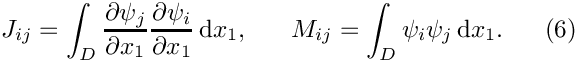. Compare the computed results to the analytic solution. What happens to the eigenvalues and eigenfunctions?